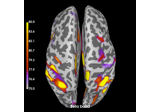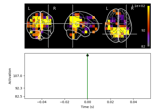# mne.connectivity.degree¶

mne.connectivity.degree(connectivity, threshold_prop=0.2)[source]

Compute the undirected degree of a connectivity matrix.

Parameters
connectivity`ndarray`, shape (n_nodes, n_nodes)

The connectivity matrix.

threshold_prop`float`

The proportion of edges to keep in the graph before computing the degree. The value should be between 0 and 1.

Returns
degree`ndarray`, shape (n_nodes,)

The computed degree.

Notes

During thresholding, the symmetry of the connectivity matrix is auto-detected based on `numpy.allclose()` of it with its transpose.

## Examples using `mne.connectivity.degree`¶